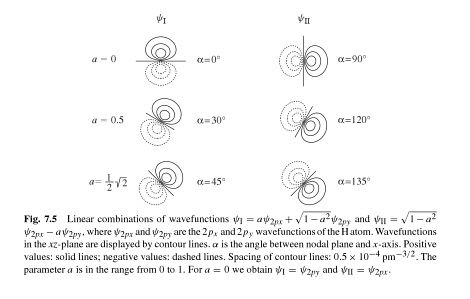# How to prove that the orientation of the atomic orbitals in the superposition $\psi= a\psi_{1} + b\psi_{2}$depends on the coefficients $a$ & $b$?

Hybridisation is a quantum superposition of orthogonal atomic orbitals; for instance $$\newcommand{\k}{\langle #1 \lvert #2 \rangle} \psi_{sp^3}= c_1\psi_{2s}+ c_2\psi_{2p_{x}} + c_3\psi_{2p_y} + c_4\psi_{2p_{z}}.$$

It is a quantum superposition which implies the coefficients $c$ to be the probability amplitudes for finding the electron in respective atomic orbital provided that it is prepared in $\lvert\psi_{sp^3}\rangle$(provided $\sum_{i=1}^4 c_1^2 =1$) that is, $$c_1= \k{\psi_{2s}}{\psi_{sp^3}}, \\ c_2= \k{\psi_{2p_x}}{\psi_{sp^3}},\\ c_3= \k{\psi_{2p_y}}{\psi_{sp^3}},\\ c_4= \k{\psi_{2p_z}}{\psi_{sp^3}}.$$

Up to this, I could easily understand this as it was just like some other superposed state often encountered in QM like $\lvert\phi\rangle = \lvert i\rangle c_i + \lvert j\rangle c_j + \lvert k\rangle c_k$ where $c_i = \k{i}{\phi}.$

But, here in the case of hybridisation, they are much more than mere comples numbers that are the amplitudes of the electron to find it in one of the base states that is the associated atomic orbitals when being prepared at the hybridised state. It is evident from Peter Atkins' words:

The coefficients in the hybrid have been chosen to give the correct directional properties of the hybrid. The squares of the coefficients give the proportion of each atomic orbital in the hybrid.

Also, the snapshot$^1$ below shows how for different values of coefficients, the spatial orientation is changed.That means the coefficients direct the spatial orientation of the atomic orbitals that are the base states for the superposition or hybridisation.

Is there any mathematical deduction that shows the orientation of the atomic orbitals in the superposition depends on the coefficients $c_i$?

I'm basically not understanding how these coefficients, which are rather probability amplitudes, would involve in determining the spatial orientations of the atomic orbitals. Can anyone explain me why these probability amplitudes influence the orientation of the atomic orbitals? Also, what is the mathematical proof of this?

$^1$The snapshot, above, is taken from Principles of Physical Chemistry By Hans Kuhn, Horst-Dieter Försterling, David H. Waldeck.

• Do not cross-post same question on different SE sites. You already asked the question on Physics.SE, so wait for an answer there (or ask the mods there to migrate it here). Oct 12, 2015 at 10:28
• I'm already deleting it. So, no offense @Wildcat .
– user5764
Oct 12, 2015 at 10:32

In quantum mechanics states/orbitals are defined within the mathematical framework of the Hilbert space. A Hilbert space is a vector space that generalizes the notion of Euclidean space - one example of the latter would be the normal two- or three-dimensional spaces you might be used to from basic geometry. So, orbitals are nothing more but abstract vectors in a Hilbert space and you can draw many analogies between them and the more familiar two- or three dimensional vectors you learn about in high school. So, let's think of quantum mechanical states as vectors for now. If you add two vectors, $\vec{a}$ and $\vec{b}$, you get another vector, $\vec{c} = \vec{a} + \vec{b}$, which if $\vec{a}$ and $\vec{b}$ are linearly independent, will have a direction different from either $\vec{a}$ or $\vec{b}$. You can also choose different coefficients for the superposition, e.g. $1$ for $\vec{a}$ and $-1$ for $\vec{b}$ yielding a vector $\vec{d} = \vec{a} - \vec{b}$ which now has a direction different from either $\vec{a}$, $\vec{b}$ or $\vec{c}$. So, the direction of a sum vector changes with the coefficients.The same principle holds true for orbitals. If you for example think of the $\mathrm{p}_{x}$ orbital as a vector pointing in the $x$ direction, i.e. in two dimensions $\bigl(\begin{smallmatrix}1 \\ 0 \end{smallmatrix}\bigr)$, and of the $\mathrm{p}_{y}$ orbital as a vector pointing in the $y$ direction, i.e. in two dimensions $\bigl(\begin{smallmatrix}0 \\ 1 \end{smallmatrix}\bigr)$, then a superposition of both (with equal coefficients of $\frac{1}{\sqrt{2}}$ to ensure normalisation) will give you a $\mathrm{p}$ orbital pointing in the $\bigl(\begin{smallmatrix}1 \\ 1 \end{smallmatrix}\bigr)$ direction.But as with vectors you are by no means confined to simply add them together with equal coefficients. For example, if you add $2$ parts of $\mathrm{p}_{x}$ and $1$ part of $\mathrm{p}_{y}$ you get a $\mathrm{p}$ orbital pointing in the $\bigl(\begin{smallmatrix}2 \\ 1 \end{smallmatrix}\bigr)$ direction, and that is clearly different from $\bigl(\begin{smallmatrix}1 \\ 1 \end{smallmatrix}\bigr)$.

• I appreciate your effort, sir but Feynman always in his lecture cautioned that state-vectors are rather abstract stuffs & can't be comparable to three-dimensional vectors. Hmmm....Quantum superposition has no analogy with vector-addition:(
– user5764
Oct 12, 2015 at 16:17
• There certainly are differences but in your case you only need to use the basic properties that all vector spaces share and that thus allow you to make analogies from three-dimensional euclidean to hilbert spaces: for example every linear combination of members of a vector space must also be a member of the vector space. And those linear combinations can be done for euclidean and hilbert spaces alike. Also, if two members of a vector space are linearly independent then they have a different "direction". Those are general concepts that don't depend on the kind of vector space. Oct 12, 2015 at 16:28
• @user36790 Well, quantum mechanical states are complex functions rather than real vectors. That alone gives you some important differences. But I don't have any striking pitfalls in the back of my mind where it is actually dangerous to draw the analogy between three-dimensional vectors and quantum states. Oct 12, 2015 at 16:39
• @user36790 Btw, what you do when forming hybrid orbitals is not a quantum superposition but a change of basis or rotation of your coordinate system, in the same sense as that you can use the vectors $\bigl(\begin{smallmatrix}1 \\ 0 \end{smallmatrix}\bigr)$ and $\bigl(\begin{smallmatrix}0 \\ 1 \end{smallmatrix}\bigr)$ as a basis for the two-dimensional euclidean space but you can just as well use linear independent combinations of the two, e.g. ... Oct 12, 2015 at 16:51
• ... $\frac{1}{\sqrt{2}}\bigl(\begin{smallmatrix}1 \\ -1 \end{smallmatrix}\bigr)$ and $\frac{1}{\sqrt{2}} \bigl(\begin{smallmatrix}1 \\ 1 \end{smallmatrix}\bigr)$. Oct 12, 2015 at 16:51